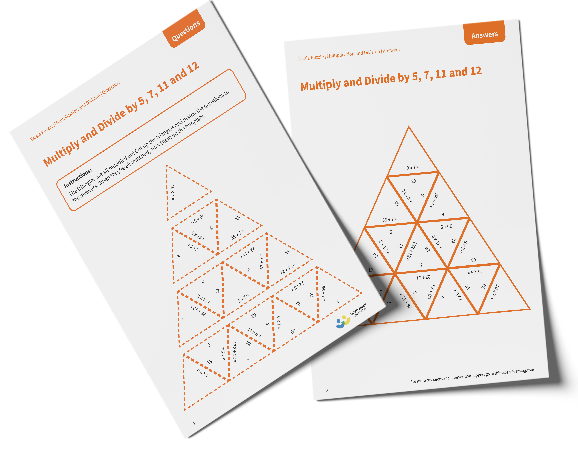# Tarsia Puzzle Multiply and Divide by 5, 7, 11 and 12

The triangles in the tarsia puzzle have all been mixed up. In this multiply and divide by 5, 7, 11 and 12 tarsia puzzle, pupils will need to match the multiplication or division question to the correct answer to create one large triangle. This worksheet would be appropriate for Year 4, Year 5 or Year 6.

This multiply and divide by 5, 7, 11 and 12 tarsia puzzle worksheet contains:

• One question sheet
• One template sheet

Year 4, Year 5, Year 6

Multiplication, Division